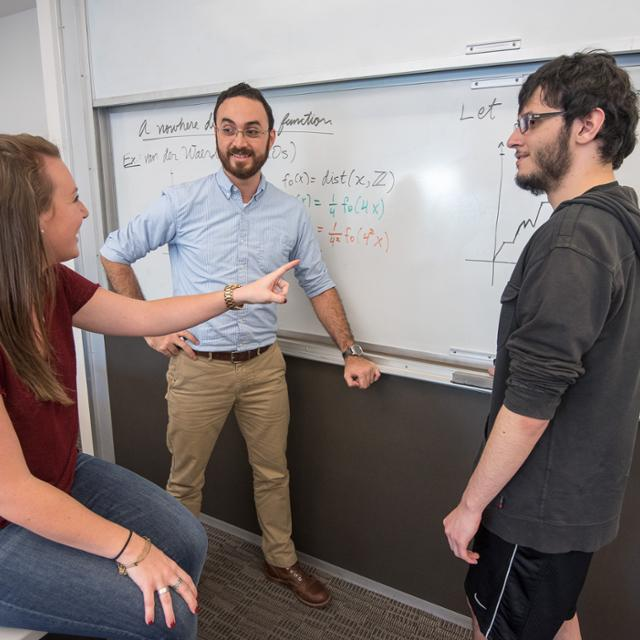# BS in MathematicsThis is the more demanding of the two undergraduate majors offered in the Department of Mathematics. This degree is appropriate for students planning to go on to graduate school in mathematics and related fields, and for anyone who wants a more challenging mathematical experience at TCU.

## Requirements for a Bachelor of Science (BS)

• MATH 10524 Calculus I
• MATH 20123 Discrete Mathematics I
• MATH 20524 Calculus II
• MATH 30053 Introduction to Mathematical Proof
• MATH 30224 Linear Algebra
• MATH 30524 Calculus III

## Students pursuing a BS degree in mathematics have a choice of two tracks:

• MATH 30803 Probability
• MATH 50253 Abstract Algebra I
•  MATH 50503 Real Analysis I

You must also must take an additional fifteen hours of mathematics courses at or above the 30000 level.

• MATH 30613 Differential Equations
• MATH 30853 Statistics

You must take an additional twelve hours of mathematics courses, including MATH 40223 or MATH 40663, from among the following:

• MATH 30803 Probability
• MATH 40103 Graph Theory
• MATH 40223 Applied Linear Algebra
• MATH 40553 Modern Fourier Analysis
• MATH 40633 Game Theory
• MATH 40643 Dynamical Systems
• MATH 40663 Numerical Analysis
• MATH 50403 Complex Analysis
• MATH 50503 Real Analysis I
• MATH 50613 Partial Differential Equations
• MATH 50623 Applied Mathematics I

Students must also take an additional six hours of mathematics courses at or above the 30000 level.

In addition, students in Track 1 or Track 2 are required to take one of the following programming courses:

• COSC 10403 Introduction to Programming
• COSC 10503 Introduction to Programming for Engineering and Science
• COSC 10603 Introduction to Python for Data Analytics
• ENGR 10573 Applied Programming Matlab

In addition, the student is required to take two of the following:

• PHYS 20474 Physics I
• PHYS 20484 Physics II
• COSC 20203 Techniques in Programming
• ECON 40313 Econometrics

Credit will not be granted for both MATH 10283 and MATH 10524. Students must earn a grade of C- or better in each mathematics course for that course to count toward a mathematics degree. Students must also have a 2.0 average or better in their mathematics courses in order to graduate with a degree in mathematics.

Students planning to do graduate work in mathematics are strongly recommended to work closely with an adviser to plan their upper-division electives.